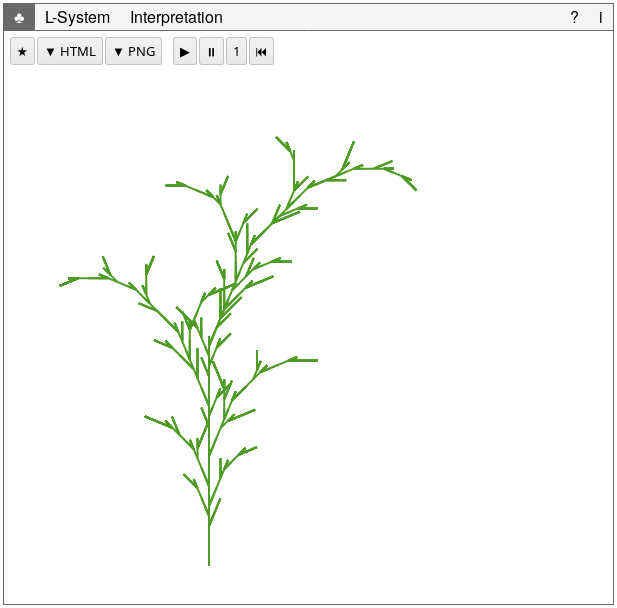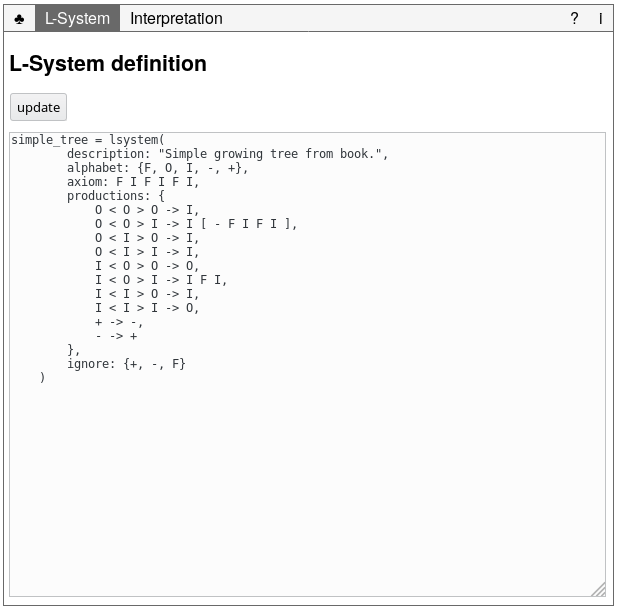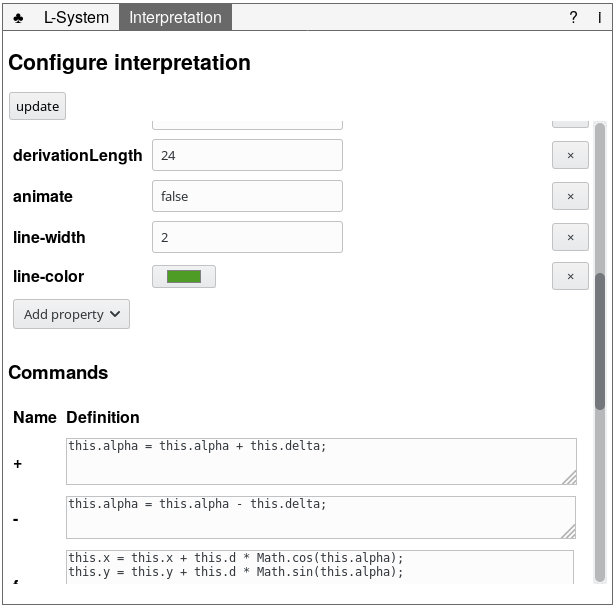# Chapter 1. Introduction

A while back I was reading Artificial life. A report from the frontier where computers meet biology [@Levy1992a]. In this book, Levy describes the development of the scientific field of artificial life till the 1990s. Although I think the book was less readable than his work Hackers [@Levy2010a], it was still an interesting read. What piqued my interest most in Levy’s book on artificial life was the idea of generating plant-like structures by means of a rewriting system known as an L-system.

In the notes to the Chapter Artificial flora, artifical fauna, artificial ecologies I found a reference to a book called The algorithmic beauty of plants [@Prusinkiewicz1990a]. Given its age, I had little hope in finding this book. To my surprise, it is available on-line! (Warning, this is a 17Mb PDF; see http://algorithmicbotany.org/papers/#abop for smaller versions.)

I downloaded the book and started reading it. Chapter 1 is introductory material introducing the L-system formalism, its extensions, and a way to render these L-systems. The authors render the L-systems by interpreting it in terms of turtle graphics. Turtle graphics is quite a simple way of thinking about drawing things with a computer: given a turtle holding a pen, tell the turtle where to move, to rotate, and to push the pen to the paper or not.

Seeing the turtle interpretation, I tried to read [@Prusinkiewicz1990a] using a constructionist approach by building the examples myself. After a couple of fun hours programming these prototypes, I decided to write my own virtual botanical laboratory because I assumed that the software described in The algorithmic beauty of plants would not exist anymore. Later, when I had finished most of the engine of my virtual botanical laboratory, I discovered that the group who put the book on-line also have put their software on-line as well.Figure 1.1 How much fun: I generated an odd looking tree!

Then again, building my own laboratory probably deepened my understanding of the material I would not have reached by using the available software. Here I present my own virtual botanical laboratory that I unoriginally named `virtual_botanical_laboratory`. Feel free to use is to explore the book The algorithmic beauty of plants yourself, use it to generate your own plant-like shapes, or just have fun with it! (For example, look at that odd looking tree I generated above :-))

In the next Chapter, the `virtual_botanical_laboratory` is introduced by enumerating the examples from Chapter 1 of The algorithmic beauty of plants defined in the `virtual_botanical_laboratory`. After that introduction, the use of the software a description of the user interface, followed by 2) an overview of the L-system language. Finally, building the software is discussed, including a list with to do items.

## 1.1 Example

How to run this example on your own is explained in the next section.

## 1.2 Running the `virtual_botanical_laboratory`

To run the `virtual_botanical_laboratory`, you need to:

• include `dist/virtual_botanical_laboratory.js` in your HTML file. For example in the HEAD like:

``````<!DOCTYPE html>
<html>
<script src="dist/virtual_botanical_laboratory.js"></script>``````
• create a LabView and configure it. For example:

``````<figure id="lab"></figure>
<script>
new virtual_botanical_laboratory.LabView("#lab", {
lsystem: `simple_tree = lsystem(
description: "Simple growing tree",
alphabet: {F, O, I, -, +},
axiom: F I F I F I,
productions: {
O < O > O -> I,
O < O > I -> I [ - F I F I ],
O < I > O -> I,
O < I > I -> I,
I < O > O -> O,
I < O > I -> I F I,
I < I > O -> I,
I < I > I -> O,
+ -> -,
- -> +
},
ignore: {+, -, F}
)`,
interpretation: {
config: {
x: 200,
y: 500,
width: 600,
height: 500,
d: 10,
delta: (-22.5 * Math.PI)/180,
alpha: (270 * Math.PI)/180,
close: false,
derivationLength: 24,
animate: false,
"line-width": 2,
"line-color": "#4E9C25"
}
}
});
</script>``````
• and open the HTML file in a modern web browser!

For more examples, please see the examples.

`virtual_botanical_laboratory` is free software; `virtual_botanical_laboratory` is released under the GPLv3. You find `virtual_botanical_laboratory`’s source code on github.

# Chapter 2. Reading The algorithmic beauty of plants

In this Chapter all examples in the first Chapter of the book The algorithmic beauty of plants, Graphical modeling using L-systems, are recreated using the `virtual_botanical_laboratory`. I recommend you read the book’s chapter while exploring the examples. In doing so, you will get familiar with the syntax of defining a L-system.

Note. Sometimes doing another derivation might crash your browser.

• Figure 1.6: Generating a quadratic Koch island [@Prusinkiewicz1990a, p. 8]Figure 2.1 The Koch island with a derivation of length 3; click to open example in the virtual_botanical_laboratory
• Figure 1.7: Examples of Koch curves generated using L-systems [@Prusinkiewicz1990a, p. 9]

•Figure 2.3 Figure 1.7.b. A quadratic modification of the snowflake curve click to open example in the virtual_botanical_laboratory
• Figure 1.8: Combination of islands and lakes [@Prusinkiewicz1990a, p. 9]Figure 2.4 Figure 1.8. Combination of islands and lakes click to open example in the virtual_botanical_laboratory
• Figure 1.9: A sequence of Koch curves obtained by successive modification of the production successor [@Prusinkiewicz1990a, p. 10]

•Figure 2.5 Figure 1.9.a: F → FF-F-F-F-F-F+F click to open example in the virtual_botanical_laboratory
•Figure 2.6 Figure 1.9.b: F → FF-F-F-F-FF click to open example in the virtual_botanical_laboratory
•Figure 2.7 Figure 1.9.c: F → FF-F+F-F-FF click to open example in the virtual_botanical_laboratory
•Figure 2.8 Figure 1.9.d: F → FF-F–F-F click to open example in the virtual_botanical_laboratory
•Figure 2.9 Figure 1.9.e: F → F-FF–F-F click to open example in the virtual_botanical_laboratory
•Figure 2.10 Figure 1.9.f: F → F-F+F-F-F click to open example in the virtual_botanical_laboratory
• Figure 1.10: Examples of curves generated by edge-rewriting L-system [@Prusinkiewicz1990a, p. 11]

•Figure 2.11 Figure 1.10.a: the dragon curve click to open example in the virtual_botanical_laboratory
• Figure 1.11: Examples of FASS curves generated by edge-rewriting L-system [@Prusinkiewicz1990a, p. 12]

•Figure 2.13 Figure 1.11.a: Hexagonal Gosper curve click to open example in the virtual_botanical_laboratory
•Figure 2.14 Figure 1.11.b: Quadratic Gosper curve or E-curve click to open example in the virtual_botanical_laboratory
• Figure 1.24: Examples of plant-like structures generated by bracketed OL-systems [@Prusinkiewicz1990a, p. 25]

•Figure 2.15 Figure 1.24.a click to open example in the virtual_botanical_laboratory
•Figure 2.16 Figure 1.24.b click to open example in the virtual_botanical_laboratory
•Figure 2.17 Figure 1.24.c click to open example in the virtual_botanical_laboratory
•Figure 2.18 Figure 1.24.d click to open example in the virtual_botanical_laboratory
•Figure 2.19 Figure 1.24.e click to open example in the virtual_botanical_laboratory
•Figure 2.20 Figure 1.24.f click to open example in the virtual_botanical_laboratory
• Figure 1.27: Stochastic branching structures [@Prusinkiewicz1990a, p. 29]Figure 2.21 Figure 1.27. Stochastic branching structures click to open example in the virtual_botanical_laboratory
• Figure 1.31: Examples of branching structures generated using L-systems based on the results of Hogeweg and Hesper [@Prusinkiewicz1990a, pp. 30---31]

•Figure 2.22 Figure 1.31.a click to open example in the virtual_botanical_laboratory
•Figure 2.23 Figure 1.31.b click to open example in the virtual_botanical_laboratory
•Figure 2.24 Figure 1.31.c click to open example in the virtual_botanical_laboratory
•Figure 2.25 Figure 1.31.d click to open example in the virtual_botanical_laboratory
•Figure 2.26 Figure 1.31.e click to open example in the virtual_botanical_laboratory
• Figure 1.37: Two curves suggesting a “row of trees” [@Prusinkiewicz1990a, p. 48]

•Figure 2.27 Figure 1.37.a click to open example in the virtual_botanical_laboratory
•Figure 2.28 Figure 1.37.b click to open example in the virtual_botanical_laboratory
• Figure 1.39: A branching pattern generated by the L-system specified in equation (1.9) [@Prusinkiewicz1990a, p. 49]

•Figure 2.29 Figure 1.39. A branching pattern generated by the L-system specified in equation (1.9) click to open example in the virtual_botanical_laboratory
•Figure 2.30 Figure 1.39. A branching pattern generated by the L-system specified in equation (1.10) click to open example in the virtual_botanical_laboratory

# Chapter 3. Using the virtual botanical laboratory

## 3.1 Interface

The `virtual_botanical_laboratory` has a very simple and bare-bones user interface. It has been added more as an afterthought than that it has been designed with user experience in mind. (Feel free to create a better interface, `virtual_botanical_laboratory` is free software after all.)

The interface is meant to show the generated images while giving the user access to the underlying L-system and the configuration of the interpretation of that L-system.

The user interface consists of five tab pages:

1. On the main tab page, labeled with , you can see the rendered L-system (see figure below). It also has some buttons to control the L-system and export the rendering.Figure 3.1 The main tab to view and control the L-system

The following “file” actions are available:

• (New): create a new empty virtual botanical laboratory in a new window.
• ▼ HTML (Export to HTML): export the virtual botanical laboratory to a HTML file. By default, this file is named after the L-system.
• ▼ PNG (Export to PNG): export the rendered L-system to a PNG file. By default, this file is named after the L-system.

The following “control” actions are available:

• ▶️ (Run): derive new successors for the L-system until it has reached the derivation length set by property `derivationLength`. You can set that option on the Interpretation tab.
• (Pause): stop deriving new successor.
• 1 (Step): derive the next successor for the L-system. Note. Due to the inefficiencies with respect to memory, this might crash your browser.
• (Reset): reset the L-system to the axiom.
2. You can view and edit the L-system definition on the L-system tab (see figure below).Figure 3.2 The L-system tab to view and change the L-system’s definition

When you change the L-system, press the Update button to have the changes take effect. This will parse the L-system’s definition. If you make an error, a warning is displayed. If everything is okay, a temporary information message to that effect is shown. Switch back to the main tab to see the changes in action.

3. You can view and change the configuration of the interpretation on the Interpretation tab (see figure below).Figure 3.3 The Interpretation tab to view and change the L-system’s interpretation

On this Interpretation tab, there are two sections:

1. Properties, which is an editable list of properties you can set, update, or remove. These properties include:

• the width and height of the canvas
• the start coordinates x, y
• the start angle alpha
• the distance to draw each `F` command, d
• the rotation to apply each `+` and `-` command, delta
• the close property to connect start and end points
• the derivationLength property to set the number or derivation steps to perform
• the animate property to indicate if each derivation step has to be shown or only the final result
• the line-width
• the line-color
2. Commands, which is a list of all commands defined in the L-system. You can edit their definitions. Note. the `this` refers to the `[Interpretation](https://heerdebeer.org/Software/virtual_botanical_laboratory/documentation/api/Interpretation.html)`.

The commands `F`, `f`, `+`, and `-` are defined by default. If you want to change their behavior, you have to introduce a new symbol in the L-system and write its command’s code. You can call the default implementation as follows:

``this.getCommand("F").execute(this);``
4. You can read a short manual on the Help tab (labeled ?).

5. You can read about the `virtual_botanical_laboratory` and its license on the About tab (labeled i).

## 3.2 The L-system language

The language to define a L-system follows the language described in the (first chapter of the) book The algorithmic beauty of plants [@Prusinkiewicz1990a]. There are some notable differences:

1. Symbol names should be separated from each other. If you want to denote an `F` followed by another `F`, write `F F` rather than `FF`.
2. Symbols can have names of any length larger than 0. So you can use symbol `Forward` instead of `F` if you so please.
3. 3D aspects of the language have not yet been implemented.
4. Language features described outside of Chapter 1 of @Prusinkiewicz1990a have not yet been implemented.

Next the features of the L-system definition language are briefly introduced. For a more thorough overview, please see the [Chapter on reading @Prusinkiewicz1990a](#reading).

### Basic L-system definitions

A basic L-system is defined by three parts:

1. An alphabet with symbols, such as `F`, `-`, and `+`.
2. The axiom or the initial string of symbols, such as `F F`.
3. A list of rewriting rules called productions, such as `F -> - F + F`.

You specify the above L-system as follows:

``````my_first_lsystem = lsystem(
alphabet: {F, -, +},
axiom: F F,
productions: {
F -> - F + F,
- -> -,
+ -> +
}
)``````

Identity rewriting rules like `+ -> +` can be omitted. If there is no rewriting rule specified for a symbol in the language, the identity rewriting rule is used by default.

For documenting purposes, you can also add a description to the L-system definition. So, the above example can be rewritten as:

``````my_first_lsystem = lsystem(
description: "This is my first L-system definition!",
alphabet: {F, -, +},
axiom: F F,
productions: {
F -> - F + F
}
)``````

One of the interesting features of L-systems are branching structures. To define a sub structure, place it in between `[` and `]`. For example:

``````expanding_tree_circle = lsystem(
alphabet: {F, -, +},
axiom: F,
productions: {
F -> F [ + F] - F
}
)``````

This will generate some expanding circle of branching lines.

### Extensions to the L-system definition language

• Stochastic L-systems. To bring some randomness into your generated plants, you can configure different successor patterns for one symbol and indicate the likelyhood these successor patterns are chosen by prepending each successor pattern with a numerical probability. The sum of these probabilities must be one (1).

For example, in the previous example you can choose circling left over right more often as follows:

``````expanding_random_tree_circle = lsystem(
alphabet: {F, -, +},
axiom: F,
productions: {
F: {
0.4 -> F [ + F] - F,
0.6 -> F [ - F] + F
}
}
)``````
• Context-aware L-system. You can choose a different successor pattern depending on the context wherein a symbol appears. For example, a `F` after a `+` can be replaced by a `f + F`, and a `F` after a `-` can be replaced by a `F - f`. You can indicate which symbols should be ignored when checking the context using the ignore keyword. For example:

``````expanding_random_tree_circle = lsystem(
alphabet: {F, f, -, +},
axiom: f,
productions: {
- < F -> F - f,
+ < F -> f + F.
f -> f [ - F] f [+ f]
},
ignore: {f}
)``````

The left context is indicated by the string before the `<` operator. You can indicate the right context by a string after the `>` operator (not shown in the example).

• Parameterized symbols. To move information through a derivation, you can user parameterized symbols. For example:

``````dx = 10;
ddx = 0.5;

expanding_random_tree_circle = lsystem(
alphabet: {F'(x), -, +},
axiom: F'(100),
productions: {
F'(x): x > 100 -> F + [ F'(x - dx) - F'(x / ddx)],
F'(x): x < 100 -> F - [ F'(x + dx) - F'(x * ddx)]
}
)``````

Here the `F'` symbol has parameter `x`. The successor to `F'(x)` differs depending on the value of `x`. These rewriting rules are conditional. You can define symbols with one or more parameters. Conditions can be complex by using Boolean operators `and`, `or`, and `not`.

Also note the definition of global values `dx` and `ddx`.

The power of parameterization is realized best by making using the parameter in the interpretation to change the behavior of the commands. For example, we can define the `F'` command as follows:

``````this.d = this.d + x;
this.getCommand("F").execute(this);``````

# Chapter 4. Developing `virtual_botanical_laboratory`

Note. The `virtual_botanical_laboratory` is quite SPACE inefficient. This is fine for the prototype it is now, but this issue needs to be addressed when continuing the project.

If you plan on extending or adapting the `virtual_botanical_laboratory`, see the API documentation.

## 4.1 Building

• To build the `virtual_botanical_laboratory`, run

``````npm install
npm run build``````
• To generate the API documentation, run

``npm run doc``
• To generate the manual you need pandocomatic and Bash, run

``./generate_documentation.sh``

## 4.2 Todo

The `virtual_botanical_laboratory` is still a work in progress. With the current version you can explore much of the material in the book The algorithmic beauty of plants [@Prusinkiewicz1990a]. Some more advanced features from later in that book needs to be implemented still.

The following list of features and improvements are still to do:

• L-system language:

• Importing other l-systems
• `&` operator
• Other stuff from later in the book
• Derive a successor in a separate (worker) thread
• Interpretation:

• Rendering 3D interpretations
• An improved user interface. The current interface is just a placeholder. Enough to configure and experiment with l-systems, but in not user-friendly. Feel free to replace it with something better, pull requests are welcome!

• make derivation and rendering cancellable.